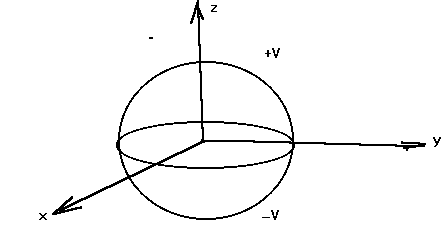# Where's the logic in this Boundary value problem?

• M. next

#### M. next

Considering the classic problem in Electrodynamics "Conducting sphere with Hemispheres at different potentials"

How does one think in order to attack this problem? I didn't get it. What potential was considered in solving this problem? Was it the +V or the -V? Or both? Why is θ' considered from 0 to ∏/2?

Here's the link: www.physics.hku.hk/~phys6503/Chapter3ZDW.ppt [Broken] (Slide number 16)

Thank you.

Last edited by a moderator:
Why make everyone download an entire ppt presentation just for a few of the slides? We are not all on cheap ultra-fast broadband :(

Please separate out what you want us to look at and put in an accessible format... you could have linked in the pic and wrote out the equations for example, or exported the slides to pdf format.

Anyway - having already been burned:
I see the problem you wanted starts at slide 17, like this:$$V(r,\theta,\varphi)=\frac{V}{4\pi}\int_0^{2\pi} d\phi^\prime \bigg[ \int_0^{\pi/2} d(-\cos\theta^\prime)-\int_{\pi/2}^\pi d(-\cos\theta^\prime) \bigg] \frac{a(r^2-a^2)}{(a^2+r^2-2ar\cos\gamma)^{3/2}}$$ note: the slide uses {} instead of [] but it does mix ##\varphi## and ##\phi## and group the integrals like that. The slide does not say what "a" is either.
What potential was considered in solving this problem? Was it the +V or the -V? Or both? Why is θ' considered from 0 to ∏/2?
... Both potentials were considered - using the superposition principle. ##\theta## is the angle to the z-axis and each hemisphere occupies 90 degrees of ##\theta##... hence the ##\pi/2##.

Put away the slide and work it out by your own favorite method, then go back and compare.

#### Attachments

Last edited:
Oh, I apologize, excuse me. Thank you for your reply. I will do.

"Conducting sphere with Hemispheres at different potentials" cannot happen.
It must be two separate hemispheres with perfect insulation between them.

It's straightforward once you get used to it mate. My professor called this the pokeball problem if I recall :)

You just need to represent the potential as a piecewise function that jumps from ##V## to ##-V## as you go from one hemisphere to another and as clem noted the hemispheres must be perfectly insulated from one another in order for such a potential difference to be maintained. You can also imagine that there is an infinitesimally small gap ##\epsilon## between the two hemispheres so that charges from one hemisphere can't flow to another; the ##\epsilon##-gap won't affect the problem because your piecewise function will now be from ##0\leq \theta< \pi/2## for ##V## and ##\pi/2< \theta \leq \pi## for ##-V## so you're only skipping a single value of the polar angle which is o.k. for integration.

The rest is just plugging in the boundary condition. The bulk of the calculations involved in this problem is extremely similar to that of the Fourier coefficients for a square wave.

Last edited: